Скачать презентацию MBA 299 Section Notes 4 18 03 Haas School

b14f92c0c8a8497d76fdc705cdaa23df.ppt

• Количество слайдов: 26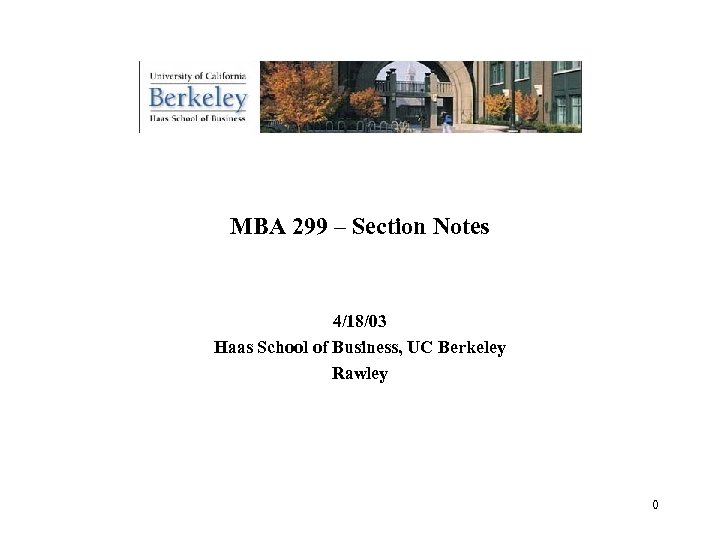MBA 299 – Section Notes 4/18/03 Haas School of Business, UC Berkeley Rawley 0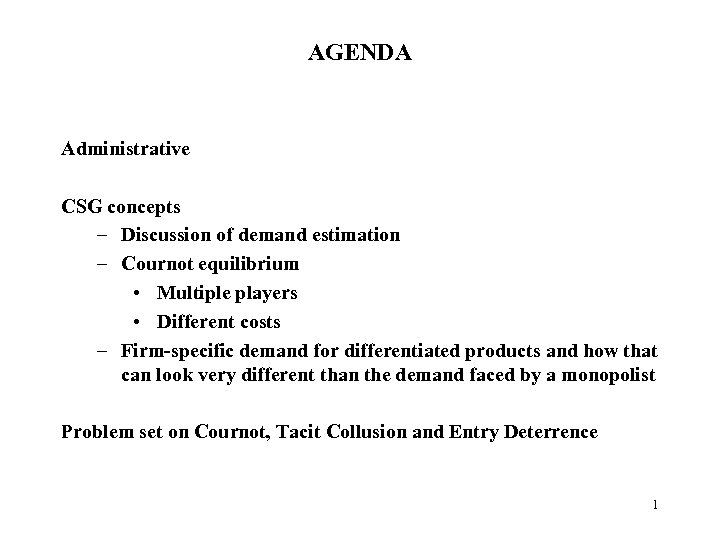AGENDA Administrative CSG concepts – Discussion of demand estimation – Cournot equilibrium • Multiple players • Different costs – Firm-specific demand for differentiated products and how that can look very different than the demand faced by a monopolist Problem set on Cournot, Tacit Collusion and Entry Deterrence 1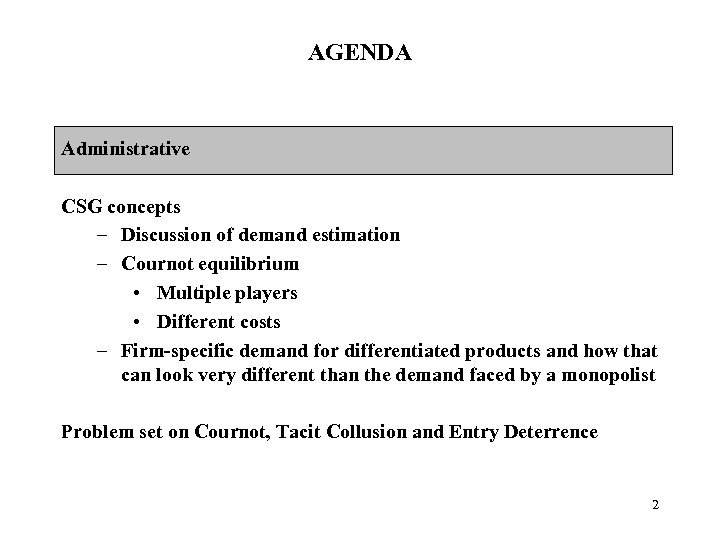AGENDA Administrative CSG concepts – Discussion of demand estimation – Cournot equilibrium • Multiple players • Different costs – Firm-specific demand for differentiated products and how that can look very different than the demand faced by a monopolist Problem set on Cournot, Tacit Collusion and Entry Deterrence 2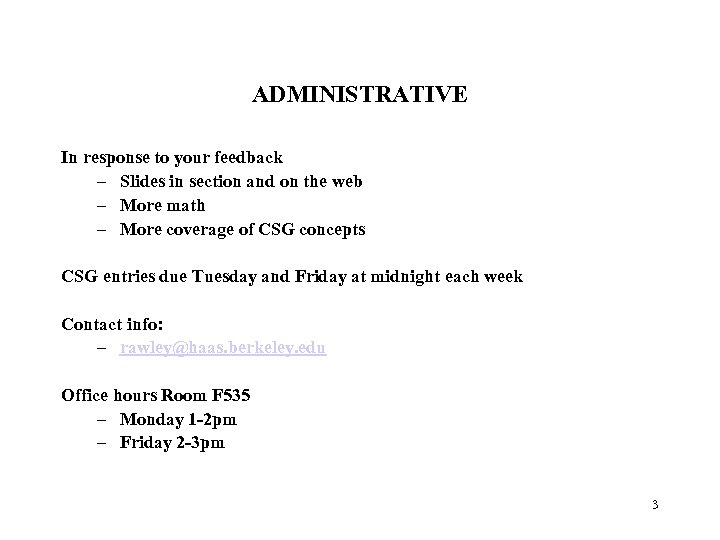ADMINISTRATIVE In response to your feedback – Slides in section and on the web – More math – More coverage of CSG concepts CSG entries due Tuesday and Friday at midnight each week Contact info: – rawley@haas. berkeley. edu Office hours Room F 535 – Monday 1 -2 pm – Friday 2 -3 pm 3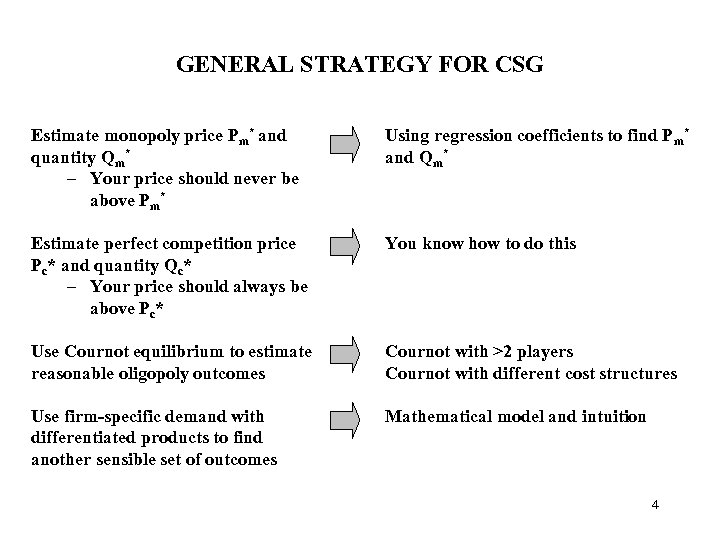GENERAL STRATEGY FOR CSG Estimate monopoly price Pm* and quantity Qm* – Your price should never be above Pm* Using regression coefficients to find Pm* and Qm* Estimate perfect competition price Pc* and quantity Qc* – Your price should always be above Pc* You know how to do this Use Cournot equilibrium to estimate reasonable oligopoly outcomes Cournot with >2 players Cournot with different cost structures Use firm-specific demand with differentiated products to find another sensible set of outcomes Mathematical model and intuition 4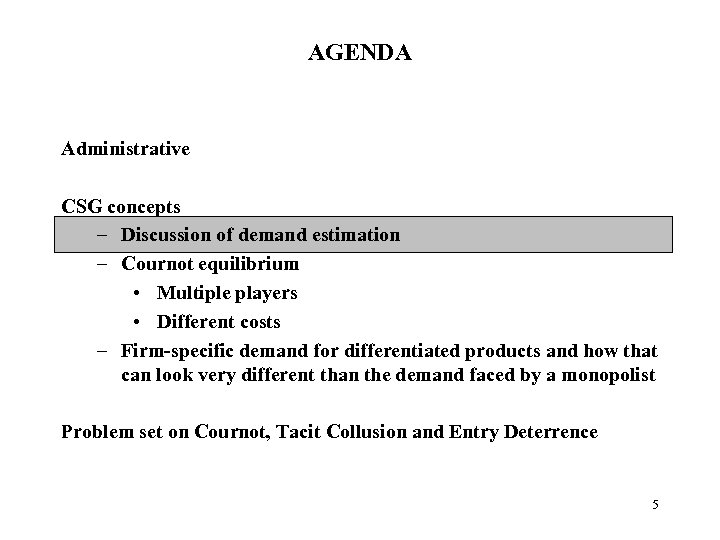AGENDA Administrative CSG concepts – Discussion of demand estimation – Cournot equilibrium • Multiple players • Different costs – Firm-specific demand for differentiated products and how that can look very different than the demand faced by a monopolist Problem set on Cournot, Tacit Collusion and Entry Deterrence 5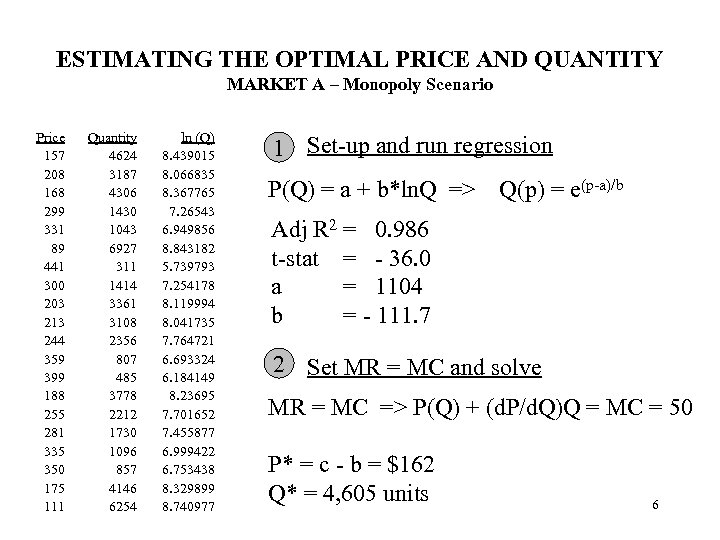ESTIMATING THE OPTIMAL PRICE AND QUANTITY MARKET A – Monopoly Scenario Price 157 208 168 299 331 89 441 300 203 213 244 359 399 188 255 281 335 350 175 111 Quantity 4624 3187 4306 1430 1043 6927 311 1414 3361 3108 2356 807 485 3778 2212 1730 1096 857 4146 6254 ln (Q) 8. 439015 8. 066835 8. 367765 7. 26543 6. 949856 8. 843182 5. 739793 7. 254178 8. 119994 8. 041735 7. 764721 6. 693324 6. 184149 8. 23695 7. 701652 7. 455877 6. 999422 6. 753438 8. 329899 8. 740977 1 Set-up and run regression P(Q) = a + b*ln. Q => Q(p) = e(p-a)/b Adj R 2 = 0. 986 t-stat = - 36. 0 a = 1104 b = - 111. 7 2 Set MR = MC and solve MR = MC => P(Q) + (d. P/d. Q)Q = MC = 50 P* = c - b = \$162 Q* = 4, 605 units 6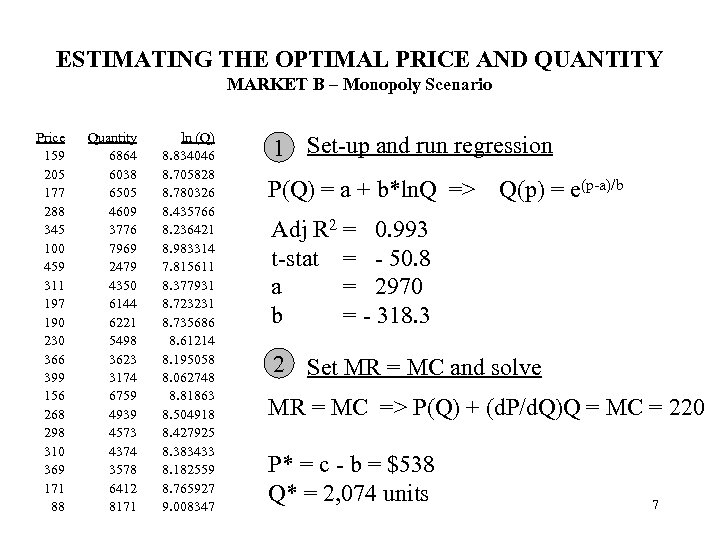ESTIMATING THE OPTIMAL PRICE AND QUANTITY MARKET B – Monopoly Scenario Price 159 205 177 288 345 100 459 311 197 190 230 366 399 156 268 298 310 369 171 88 Quantity 6864 6038 6505 4609 3776 7969 2479 4350 6144 6221 5498 3623 3174 6759 4939 4573 4374 3578 6412 8171 ln (Q) 8. 834046 8. 705828 8. 780326 8. 435766 8. 236421 8. 983314 7. 815611 8. 377931 8. 723231 8. 735686 8. 61214 8. 195058 8. 062748 8. 81863 8. 504918 8. 427925 8. 383433 8. 182559 8. 765927 9. 008347 1 Set-up and run regression P(Q) = a + b*ln. Q => Q(p) = e(p-a)/b Adj R 2 = 0. 993 t-stat = - 50. 8 a = 2970 b = - 318. 3 2 Set MR = MC and solve MR = MC => P(Q) + (d. P/d. Q)Q = MC = 220 P* = c - b = \$538 Q* = 2, 074 units 7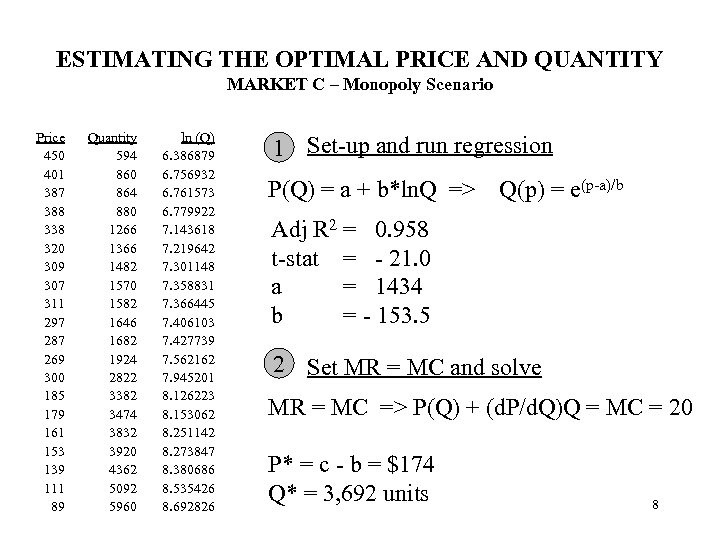ESTIMATING THE OPTIMAL PRICE AND QUANTITY MARKET C – Monopoly Scenario Price 450 401 387 388 338 320 309 307 311 297 287 269 300 185 179 161 153 139 111 89 Quantity 594 860 864 880 1266 1366 1482 1570 1582 1646 1682 1924 2822 3382 3474 3832 3920 4362 5092 5960 ln (Q) 6. 386879 6. 756932 6. 761573 6. 779922 7. 143618 7. 219642 7. 301148 7. 358831 7. 366445 7. 406103 7. 427739 7. 562162 7. 945201 8. 126223 8. 153062 8. 251142 8. 273847 8. 380686 8. 535426 8. 692826 1 Set-up and run regression P(Q) = a + b*ln. Q => Q(p) = e(p-a)/b Adj R 2 = 0. 958 t-stat = - 21. 0 a = 1434 b = - 153. 5 2 Set MR = MC and solve MR = MC => P(Q) + (d. P/d. Q)Q = MC = 20 P* = c - b = \$174 Q* = 3, 692 units 8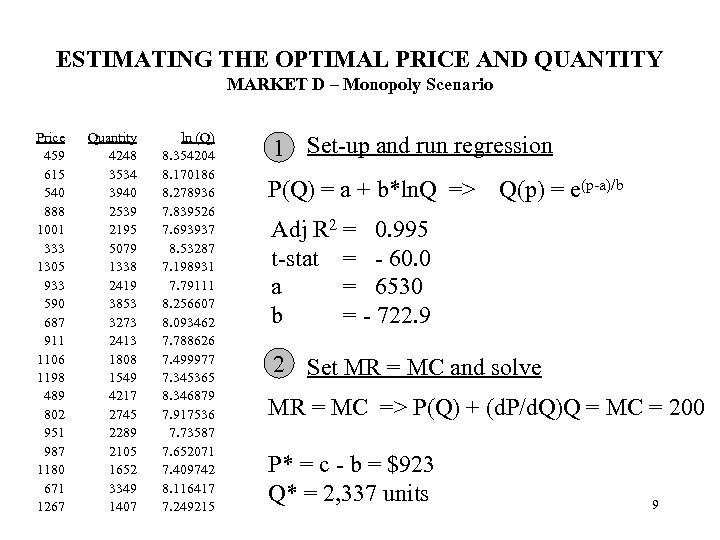ESTIMATING THE OPTIMAL PRICE AND QUANTITY MARKET D – Monopoly Scenario Price 459 615 540 888 1001 333 1305 933 590 687 911 1106 1198 489 802 951 987 1180 671 1267 Quantity 4248 3534 3940 2539 2195 5079 1338 2419 3853 3273 2413 1808 1549 4217 2745 2289 2105 1652 3349 1407 ln (Q) 8. 354204 8. 170186 8. 278936 7. 839526 7. 693937 8. 53287 7. 198931 7. 79111 8. 256607 8. 093462 7. 788626 7. 499977 7. 345365 8. 346879 7. 917536 7. 73587 7. 652071 7. 409742 8. 116417 7. 249215 1 Set-up and run regression P(Q) = a + b*ln. Q => Q(p) = e(p-a)/b Adj R 2 = 0. 995 t-stat = - 60. 0 a = 6530 b = - 722. 9 2 Set MR = MC and solve MR = MC => P(Q) + (d. P/d. Q)Q = MC = 200 P* = c - b = \$923 Q* = 2, 337 units 9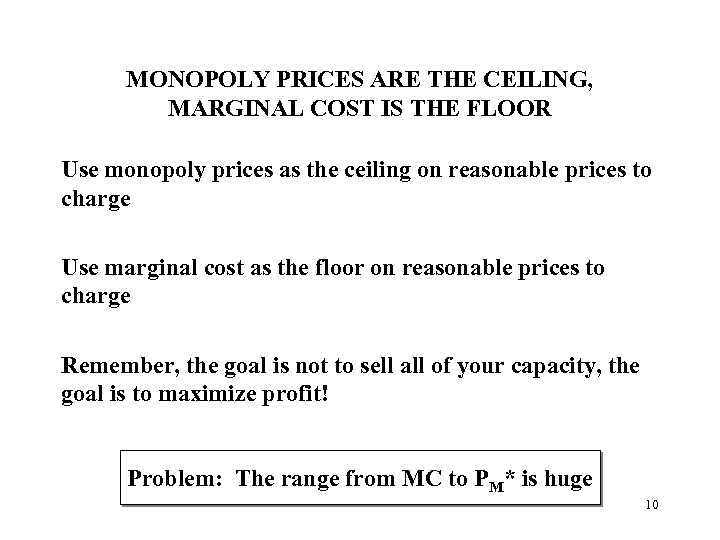MONOPOLY PRICES ARE THE CEILING, MARGINAL COST IS THE FLOOR Use monopoly prices as the ceiling on reasonable prices to charge Use marginal cost as the floor on reasonable prices to charge Remember, the goal is not to sell all of your capacity, the goal is to maximize profit! Problem: The range from MC to PM* is huge 10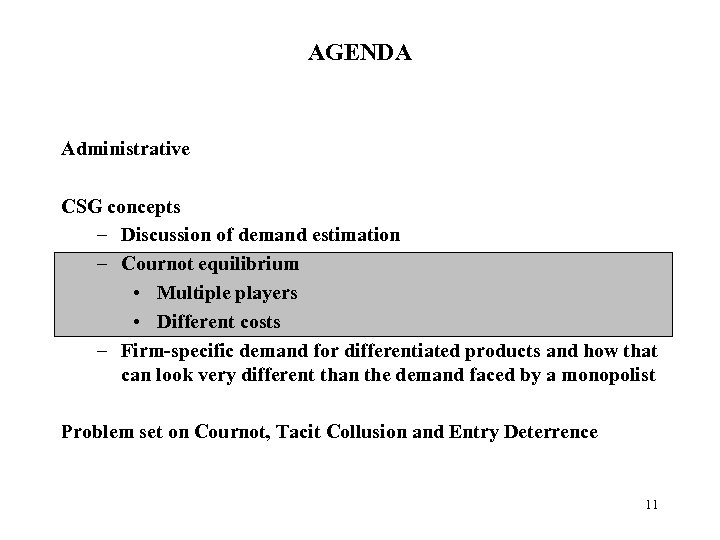AGENDA Administrative CSG concepts – Discussion of demand estimation – Cournot equilibrium • Multiple players • Different costs – Firm-specific demand for differentiated products and how that can look very different than the demand faced by a monopolist Problem set on Cournot, Tacit Collusion and Entry Deterrence 11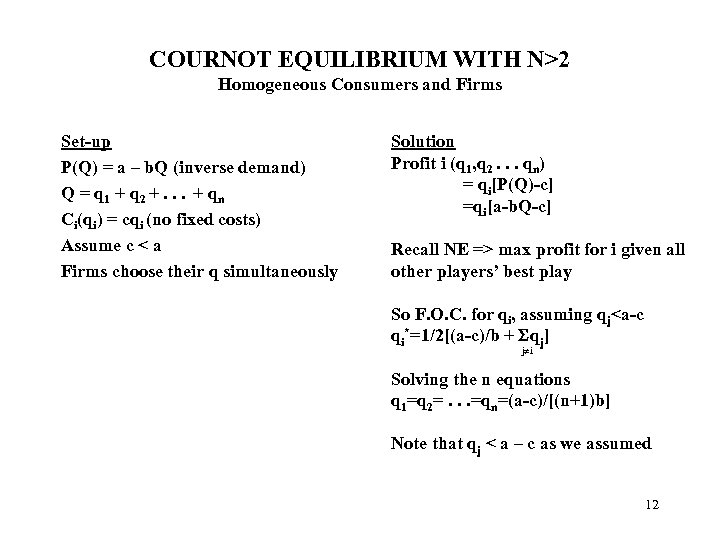COURNOT EQUILIBRIUM WITH N>2 Homogeneous Consumers and Firms Set-up P(Q) = a – b. Q (inverse demand) Q = q 1 + q 2 +. . . + qn Ci(qi) = cqi (no fixed costs) Assume c < a Firms choose their q simultaneously Solution Profit i (q 1, q 2. . . qn) = qi[P(Q)-c] =qi[a-b. Q-c] Recall NE => max profit for i given all other players’ best play So F. O. C. for qi, assuming qj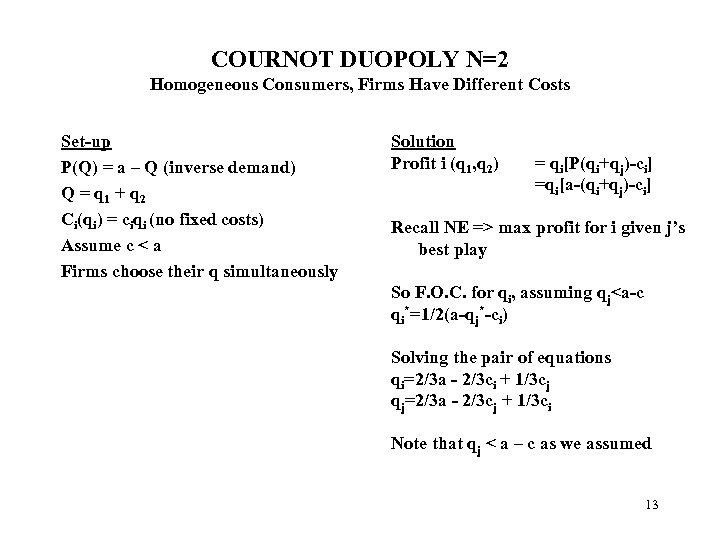COURNOT DUOPOLY N=2 Homogeneous Consumers, Firms Have Different Costs Set-up P(Q) = a – Q (inverse demand) Q = q 1 + q 2 Ci(qi) = ciqi (no fixed costs) Assume c < a Firms choose their q simultaneously Solution Profit i (q 1, q 2) = qi[P(qi+qj)-ci] =qi[a-(qi+qj)-ci] Recall NE => max profit for i given j’s best play So F. O. C. for qi, assuming qj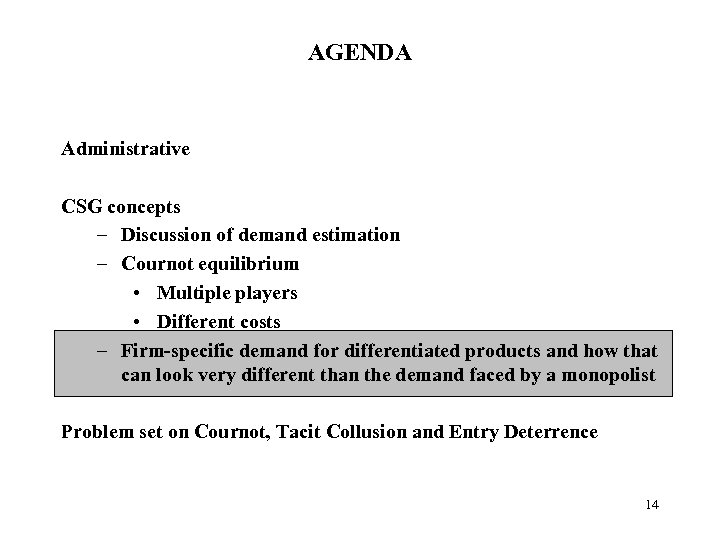AGENDA Administrative CSG concepts – Discussion of demand estimation – Cournot equilibrium • Multiple players • Different costs – Firm-specific demand for differentiated products and how that can look very different than the demand faced by a monopolist Problem set on Cournot, Tacit Collusion and Entry Deterrence 14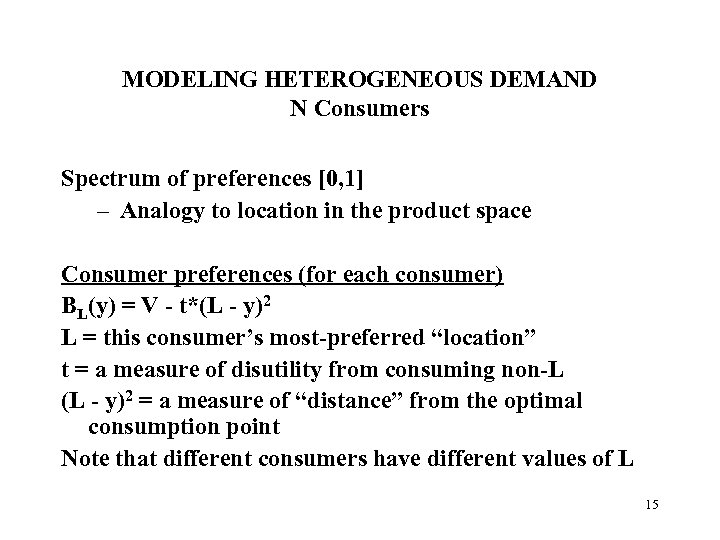MODELING HETEROGENEOUS DEMAND N Consumers Spectrum of preferences [0, 1] – Analogy to location in the product space Consumer preferences (for each consumer) BL(y) = V - t*(L - y)2 L = this consumer’s most-preferred “location” t = a measure of disutility from consuming non-L (L - y)2 = a measure of “distance” from the optimal consumption point Note that different consumers have different values of L 15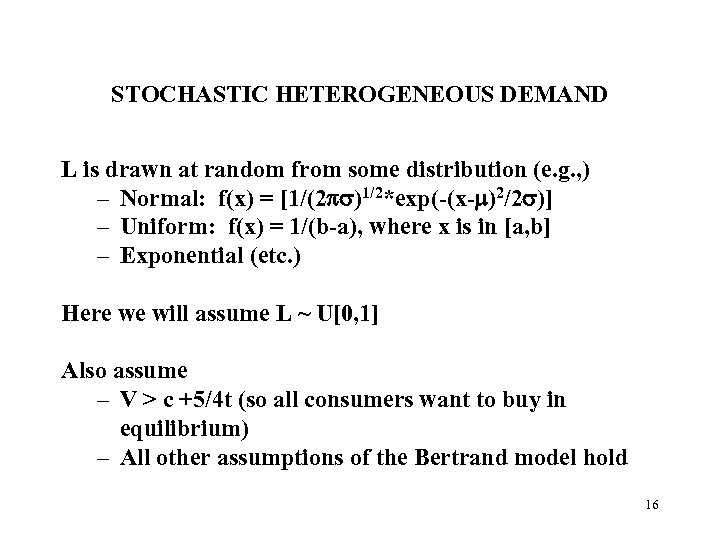STOCHASTIC HETEROGENEOUS DEMAND L is drawn at random from some distribution (e. g. , ) – Normal: f(x) = [1/(2 )1/2*exp(-(x- )2/2 )] – Uniform: f(x) = 1/(b-a), where x is in [a, b] – Exponential (etc. ) Here we will assume L ~ U[0, 1] Also assume – V > c +5/4 t (so all consumers want to buy in equilibrium) – All other assumptions of the Bertrand model hold 16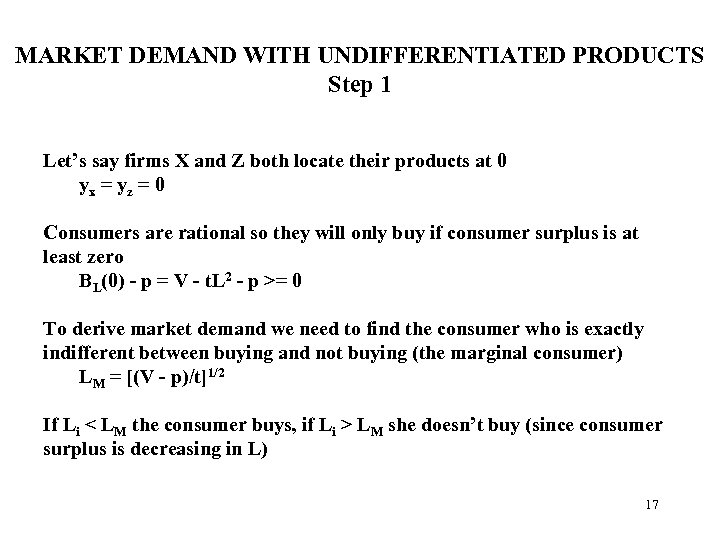MARKET DEMAND WITH UNDIFFERENTIATED PRODUCTS Step 1 Let’s say firms X and Z both locate their products at 0 yx = yz = 0 Consumers are rational so they will only buy if consumer surplus is at least zero BL(0) - p = V - t. L 2 - p >= 0 To derive market demand we need to find the consumer who is exactly indifferent between buying and not buying (the marginal consumer) LM = [(V - p)/t]1/2 If Li < LM the consumer buys, if Li > LM she doesn’t buy (since consumer surplus is decreasing in L) 17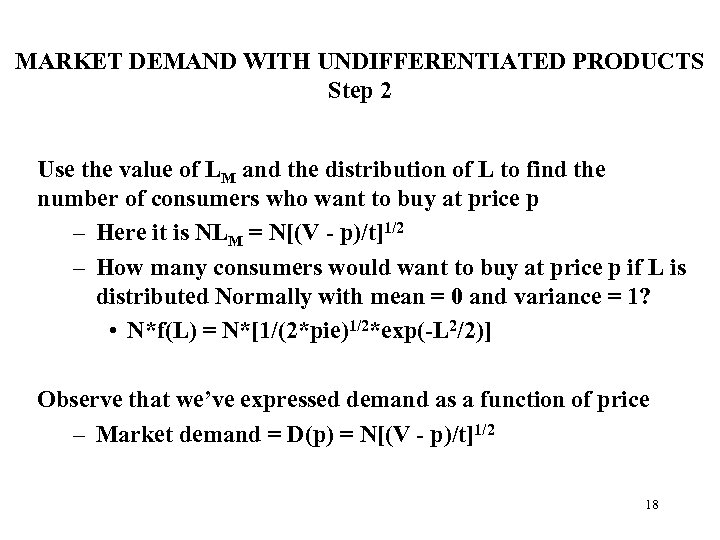MARKET DEMAND WITH UNDIFFERENTIATED PRODUCTS Step 2 Use the value of LM and the distribution of L to find the number of consumers who want to buy at price p – Here it is NLM = N[(V - p)/t]1/2 – How many consumers would want to buy at price p if L is distributed Normally with mean = 0 and variance = 1? • N*f(L) = N*[1/(2*pie)1/2*exp(-L 2/2)] Observe that we’ve expressed demand as a function of price – Market demand = D(p) = N[(V - p)/t]1/2 18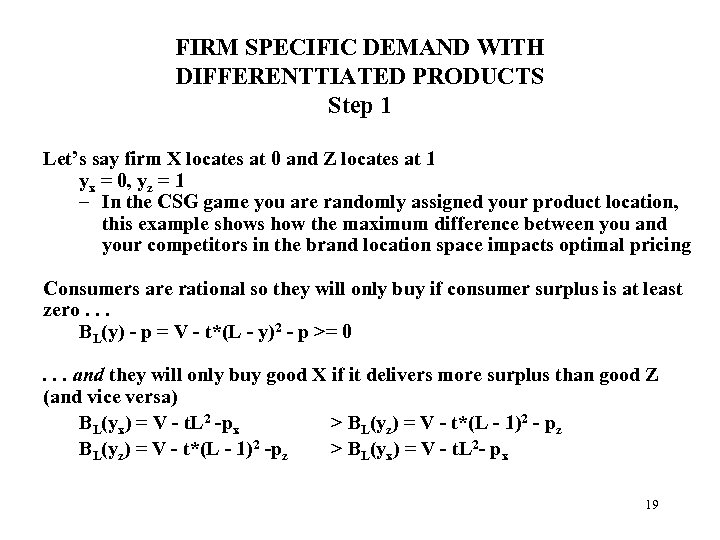FIRM SPECIFIC DEMAND WITH DIFFERENTTIATED PRODUCTS Step 1 Let’s say firm X locates at 0 and Z locates at 1 yx = 0, yz = 1 – In the CSG game you are randomly assigned your product location, this example shows how the maximum difference between you and your competitors in the brand location space impacts optimal pricing Consumers are rational so they will only buy if consumer surplus is at least zero. . . BL(y) - p = V - t*(L - y)2 - p >= 0. . . and they will only buy good X if it delivers more surplus than good Z (and vice versa) BL(yx) = V - t. L 2 -px > BL(yz) = V - t*(L - 1)2 - pz BL(yz) = V - t*(L - 1)2 -pz > BL(yx) = V - t. L 2 - px 19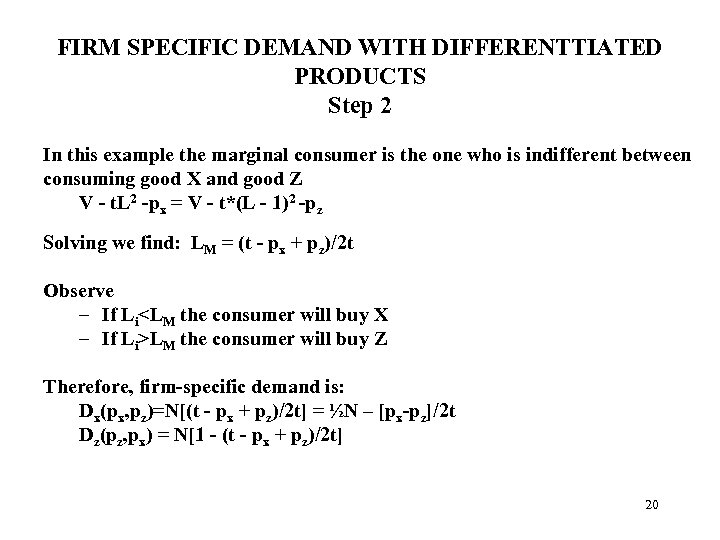FIRM SPECIFIC DEMAND WITH DIFFERENTTIATED PRODUCTS Step 2 In this example the marginal consumer is the one who is indifferent between consuming good X and good Z V - t. L 2 -px = V - t*(L - 1)2 -pz Solving we find: LM = (t - px + pz)/2 t Observe – If LiLM the consumer will buy Z Therefore, firm-specific demand is: Dx(px, pz)=N[(t - px + pz)/2 t] = ½N – [px-pz]/2 t Dz(pz, px) = N[1 - (t - px + pz)/2 t] 20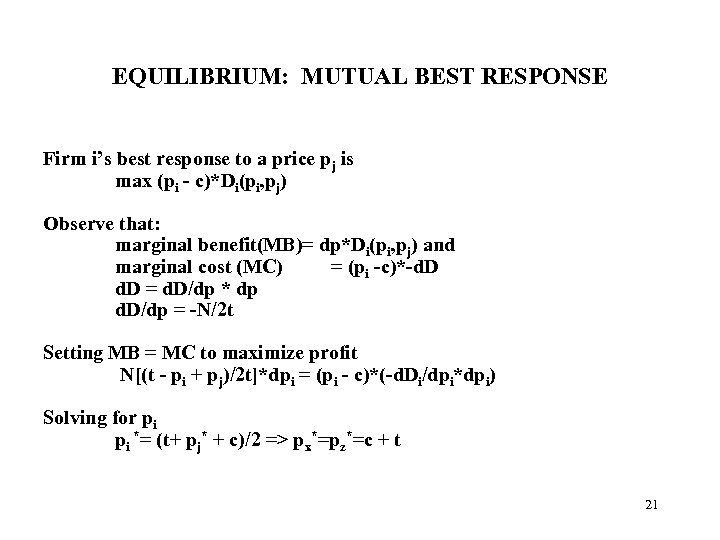EQUILIBRIUM: MUTUAL BEST RESPONSE Firm i’s best response to a price pj is max (pi - c)*Di(pi, pj) Observe that: marginal benefit(MB)= dp*Di(pi, pj) and marginal cost (MC) = (pi -c)*-d. D = d. D/dp * dp d. D/dp = -N/2 t Setting MB = MC to maximize profit N[(t - pi + pj)/2 t]*dpi = (pi - c)*(-d. Di/dpi*dpi) Solving for pi pi *= (t+ pj* + c)/2 => px*=pz*=c + t 21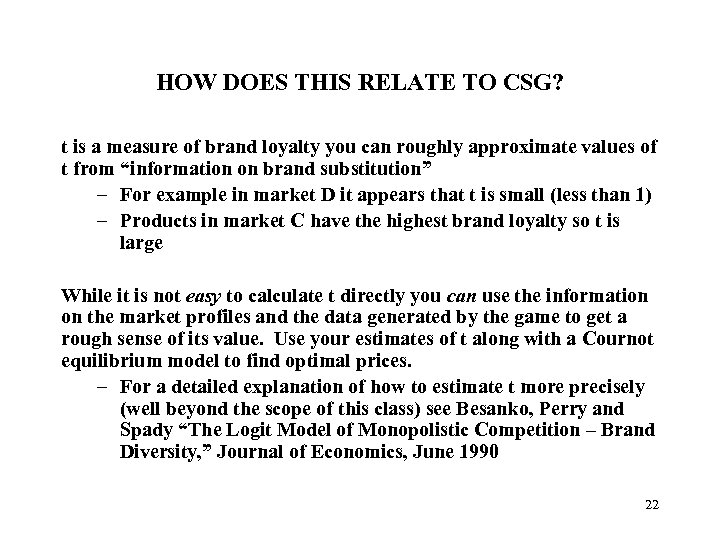HOW DOES THIS RELATE TO CSG? t is a measure of brand loyalty you can roughly approximate values of t from “information on brand substitution” – For example in market D it appears that t is small (less than 1) – Products in market C have the highest brand loyalty so t is large While it is not easy to calculate t directly you can use the information on the market profiles and the data generated by the game to get a rough sense of its value. Use your estimates of t along with a Cournot equilibrium model to find optimal prices. – For a detailed explanation of how to estimate t more precisely (well beyond the scope of this class) see Besanko, Perry and Spady “The Logit Model of Monopolistic Competition – Brand Diversity, ” Journal of Economics, June 1990 22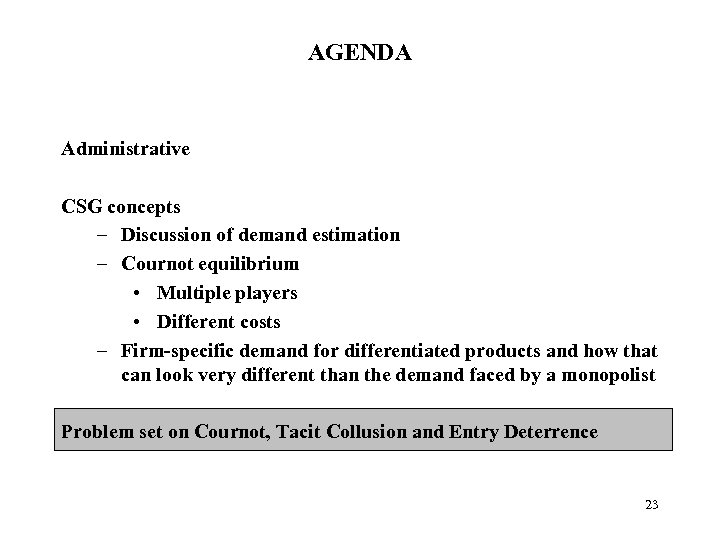AGENDA Administrative CSG concepts – Discussion of demand estimation – Cournot equilibrium • Multiple players • Different costs – Firm-specific demand for differentiated products and how that can look very different than the demand faced by a monopolist Problem set on Cournot, Tacit Collusion and Entry Deterrence 23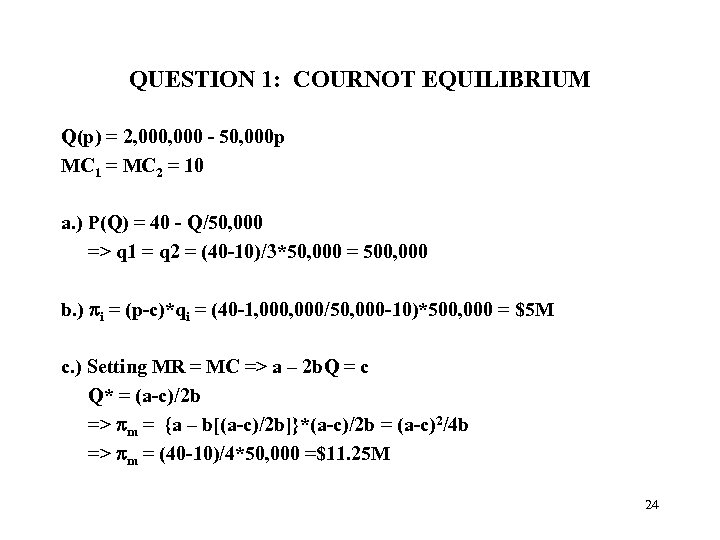QUESTION 1: COURNOT EQUILIBRIUM Q(p) = 2, 000 - 50, 000 p MC 1 = MC 2 = 10 a. ) P(Q) = 40 - Q/50, 000 => q 1 = q 2 = (40 -10)/3*50, 000 = 500, 000 b. ) i = (p-c)*qi = (40 -1, 000/50, 000 -10)*500, 000 = \$5 M c. ) Setting MR = MC => a – 2 b. Q = c Q* = (a-c)/2 b => m = {a – b[(a-c)/2 b]}*(a-c)/2 b = (a-c)2/4 b => m = (40 -10)/4*50, 000 =\$11. 25 M 24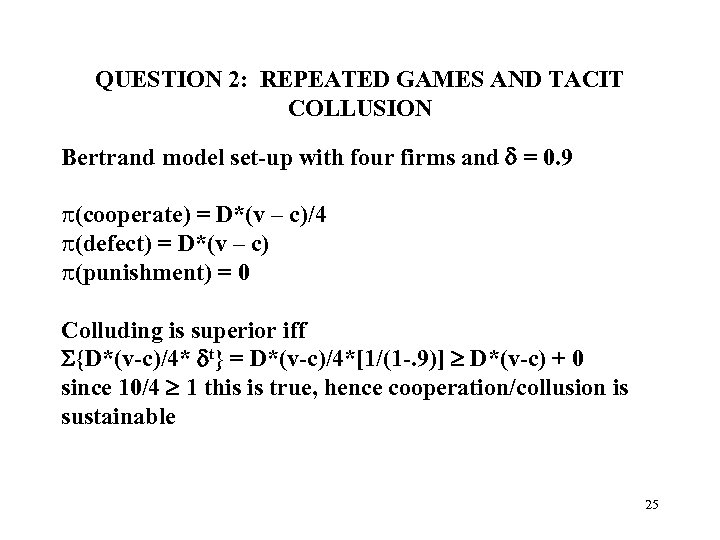QUESTION 2: REPEATED GAMES AND TACIT COLLUSION Bertrand model set-up with four firms and = 0. 9 p(cooperate) = D*(v – c)/4 p(defect) = D*(v – c) p(punishment) = 0 Colluding is superior iff {D*(v-c)/4* t} = D*(v-c)/4*[1/(1 -. 9)] D*(v-c) + 0 since 10/4 1 this is true, hence cooperation/collusion is sustainable 25